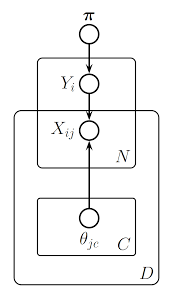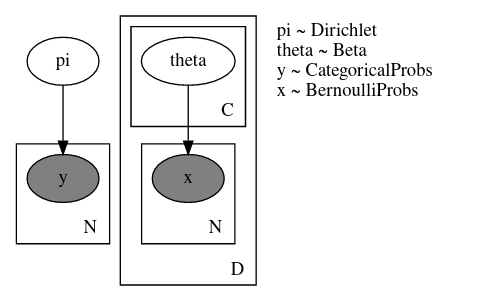# Numpyro.plate dim - Bayesian Naive Bayes

Hi all,

I am trying to reproduce a Bayesian naive Bayes model from the plate diagram in Kevin Murphy’s Machine Learning: A probabilistic Perspective 2012, Chapter 10, Section 10.4.1, Figure 10.8b, page 322. The figure is taken from the course Probabilistic Graphical Models, slide 16 of Lecture 2: Directed Graphical Models:I got the model to work on a toy dataset, compared the results to scikit-learn’s `BernoulliNB`. The model seems to recover the parameters from the toy data, and I have no divergences or any `r_hat > 1`. The complete code here, and the minimal code for the model is below.

Minimal code, working example
``````import jax
import jax.numpy as jnp

import numpyro
import numpyro.distributions as dist
from numpyro.infer import MCMC, NUTS

key = jax.random.PRNGKey(0)
key

keys = jax.random.split(key, 2)
d_X = dist.Bernoulli(jnp.array((0.91, 0.02, 0.90, 0.32, 0.66, 0.71, 0.32, 0.70, 0.94, 0.49)))
d_y = dist.Bernoulli(jnp.array((0.8)))
X = d_X.sample(keys, (300,))
y = d_y.sample(keys, (300,))

def model(X, num_classes, y=None,):
num_items, num_features = X.shape
pi = numpyro.sample("pi", dist.Dirichlet(jnp.ones(num_classes)))

with numpyro.plate("D", num_features, dim=None):
with numpyro.plate("C", num_classes):
theta = numpyro.sample("theta", dist.Beta(1,1))

with numpyro.plate("N", num_items, dim=-2):
y = numpyro.sample("y", dist.Categorical(pi), obs=y)
with numpyro.plate("D", num_features, dim=None):
x = numpyro.sample("x", dist.Bernoulli(theta[y]), obs=X)

nuts_kernel = NUTS(model)
mcmc = MCMC(nuts_kernel, num_warmup=500, num_samples=1000, num_chains=4)
mcmc.run(key, X, 2, y, extra_fields=("potential_energy",))
mcmc.print_summary()
``````

Output:

``````sample: 100%|██████████| 1500/1500 [00:11<00:00, 129.06it/s, 7 steps of size 3.95e-01. acc. prob=0.87]
sample: 100%|██████████| 1500/1500 [00:06<00:00, 246.59it/s, 15 steps of size 4.08e-01. acc. prob=0.86]
sample: 100%|██████████| 1500/1500 [00:05<00:00, 270.71it/s, 7 steps of size 4.91e-01. acc. prob=0.85]
sample: 100%|██████████| 1500/1500 [00:06<00:00, 240.69it/s, 7 steps of size 4.40e-01. acc. prob=0.86]
mean       std    median      5.0%     95.0%     n_eff     r_hat
pi      0.17      0.00      0.17      0.17      0.18   6765.86      1.00
pi      0.83      0.00      0.83      0.82      0.83   6766.00      1.00
theta[0,0]      0.85      0.05      0.86      0.77      0.93   5084.27      1.00
theta[0,1]      0.06      0.03      0.05      0.01      0.10   6469.38      1.00
theta[0,2]      0.89      0.04      0.89      0.82      0.96   4864.51      1.00
theta[0,3]      0.28      0.06      0.27      0.18      0.38   5242.58      1.00
theta[0,4]      0.67      0.06      0.67      0.56      0.77   4682.34      1.00
theta[0,5]      0.69      0.06      0.69      0.58      0.79   5221.52      1.00
theta[0,6]      0.30      0.06      0.29      0.19      0.40   4822.39      1.00
theta[0,7]      0.61      0.07      0.61      0.51      0.72   5376.68      1.00
theta[0,8]      0.89      0.04      0.89      0.82      0.95   5293.73      1.00
theta[0,9]      0.59      0.06      0.59      0.49      0.70   5938.99      1.00
theta[1,0]      0.91      0.02      0.91      0.88      0.94   5269.80      1.00
theta[1,1]      0.01      0.01      0.01      0.00      0.02   5056.84      1.00
theta[1,2]      0.90      0.02      0.90      0.86      0.93   5359.81      1.00
theta[1,3]      0.36      0.03      0.36      0.30      0.41   6750.08      1.00
theta[1,4]      0.66      0.03      0.66      0.61      0.71   4756.77      1.00
theta[1,5]      0.68      0.03      0.68      0.63      0.73   5011.69      1.00
theta[1,6]      0.32      0.03      0.32      0.28      0.37   4942.66      1.00
theta[1,7]      0.69      0.03      0.69      0.64      0.73   5178.98      1.00
theta[1,8]      0.94      0.01      0.95      0.92      0.97   5130.13      1.00
theta[1,9]      0.46      0.03      0.46      0.41      0.51   4781.71      1.00

Number of divergences: 0
``````

What is troubling me is my misunderstanding behind `dim` within `numpyro.plate`. It took me several iterations of trial and error to find the correct arguments of `dim` for each plate. Unfortunately, this might be convoluted with my model definition because I am repeating the plate over `D` for X_{ij}.

My misunderstanding of dimensions:

1. I would expect the shape of `theta` to be (10,2) because the first plate, `D` was given `dim=None` therefore uses the leftmost dimension, yet the `theta` has shape (2,10)
2. I don’t understand why the two plates `N` and `D` only work with `dim=-2` and `dim=None`, respectfully.

My explanation for why `dim=-2` and `dim=None` for plates `N` and `D`, respectfully, work:
Using `dim=-2` and `dim=None` (same as indexing 0?) for plate `N` and plate `D`, respectfully, I am indexing the number of rows in my 2d training data, then indexing the columns of my training data.

Yet, when I use `dim=None` and `dim=-1` for plates `N` and `D`, respectfully, I get an assertion error (shown below)? My expectation is `dim=None` and `dim=-1` is indexing the leftmost dimension for plate `N` (the rows) and rightmost dimension for plate `D` (the columns)

AssertionError
``````def model(X, num_classes, y=None,):
num_items, num_features = X.shape
pi = numpyro.sample("pi", dist.Dirichlet(jnp.ones(num_classes)))

with numpyro.plate("D", num_features, dim=None):
with numpyro.plate("C", num_classes, dim=None):
theta = numpyro.sample("theta", dist.Beta(1,1))
print(f"{theta.shape=}")

with numpyro.plate("N", num_items, dim=None):
y = numpyro.sample("y", dist.Categorical(pi), obs=y)
print(f"{y.shape=}")
with numpyro.plate("D", num_features, dim=-1):
print(f"{theta[y].shape=}")
print(f"{X.shape=}")
x = numpyro.sample("x", dist.Bernoulli(theta[y]), obs=X)
print(f"{x.shape=}")

with numpyro.handlers.seed(rng_seed=0):
for _ in range(1):
model(X_train, 2, y_train)
``````

Output:

``````theta.shape=(2, 10)
y.shape=(5,)
---------------------------------------------------------------------------
AssertionError                            Traceback (most recent call last)
<ipython-input-41-39648db3380c> in <module>
20 with numpyro.handlers.seed(rng_seed=0):
21     for _ in range(1):
---> 22         model(X_train, 2, y_train)

<ipython-input-41-39648db3380c> in model(X, num_classes, y)
11         y = numpyro.sample("y", dist.Categorical(pi), obs=y)
12         print(f"{y.shape=}")
---> 13         with numpyro.plate("D", num_features, dim=-1):
14             print(f"{theta[y].shape=}")
15             print(f"{X.shape=}")

~/anaconda3/envs/numpyro_play/lib/python3.8/site-packages/numpyro/primitives.py in __init__(self, name, size, subsample_size, dim)
401         if dim is not None and dim >= 0:
402             raise ValueError("dim arg must be negative.")
--> 403         self.dim, self._indices = self._subsample(
404             self.name, self.size, subsample_size, dim
405         )

~/anaconda3/envs/numpyro_play/lib/python3.8/site-packages/numpyro/primitives.py in _subsample(name, size, subsample_size, dim)
442             dim = new_dim
443         else:
--> 444             assert dim not in occupied_dims
445         return dim, subsample
446

AssertionError:
``````

Last possibly, unrelated question:
3. Is there a better way to index using plates to achieve the exact diagram shown in Figure 10.8(b)? I feel like it’s hacky with reusing plate `D`. The render numpryo model, below:Oops, sorry, the doc is wrong. It should be `rightmost` rather than `leftmost`… Do you want to submit a fix?

reusing plate `D`

You can do

``````def model():
plate_D = numpyro.plate("D", size, dim=...)
with plate_D:
...
# reuse the plate
with plate_D:
...
``````

achieve the exact diagram

It seems to me that the two diagrams are similar. Could you elaborate where is the difference?

It should be `rightmost` rather than `leftmost`

Oh… Yeah, that makes a lot more sense. I think I noticed this when I was stepping through the debugger. I will submit the PR shortly.

Let me double check my understanding:

``````    with numpyro.plate("D", num_features, dim=None):
with numpyro.plate("C", num_classes):
theta = numpyro.sample("theta", dist.Beta(1,1))
``````

Above is construction a tensor of rank 2 with the rightmost dimension index by plate `D` of size, `num_features`, then because nesting it moves over one dimension from the right, with a size of `num_classes` resulting in `theta.shape == (num_classes, num_features)`

`plate_D = numpyro.plate("D", size, dim=...)`

I cannot believe I didn’t think of that, haha.

Could you elaborate where is the difference?

I guess I was expecting a one-for-one output such that `numpyro.render` would overlay the plate nesting between plate `N` for variable `y` and `x`.

Since it’s not one-to-one it makes me feel like I could rewrite this model such that I don’t reuse `plate_D` to get `numpyro.render` to show a similar overlay plate between ‘y’ and ‘x’. Maybe this is a little naive.